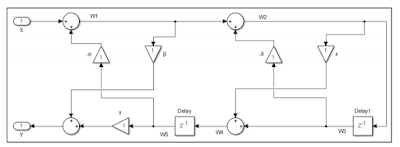## Pages

### Digital filter algorithms

In both recursive and non-recursive (FIR full form and IIR full form filter) in digital signal possessing, the algorithm can be implemented is based on DFT meaning or differential equation.

The algorithm would be used in filtering application in two specific areas like: in filtering algorithms and in signal analysis algorithms.

Their implementation is possible by using hardware or some software. Filtering algorithm can be implemented software like Matlab.

The figure below represents the diagram of the cascaded filter structure. It will help to better to understand how the comparability condition to be work.Cascaded filter design
In both recursive and non-recursive (FIR full form and IIR full form filter) in digital signal possessing, the algorithm can be implemented is based on DFT meaning or differential equation.

The algorithm would be used in filtering application in two specific areas like: in filtering algorithms and in signal analysis algorithms.

Their implementation is possible by using hardware or some software. Filtering algorithm can be implemented software like Matlab.

The figure below represents the diagram of the cascaded filter structure. It will help to better to understand how the comparability condition to be work.Cascaded filter design